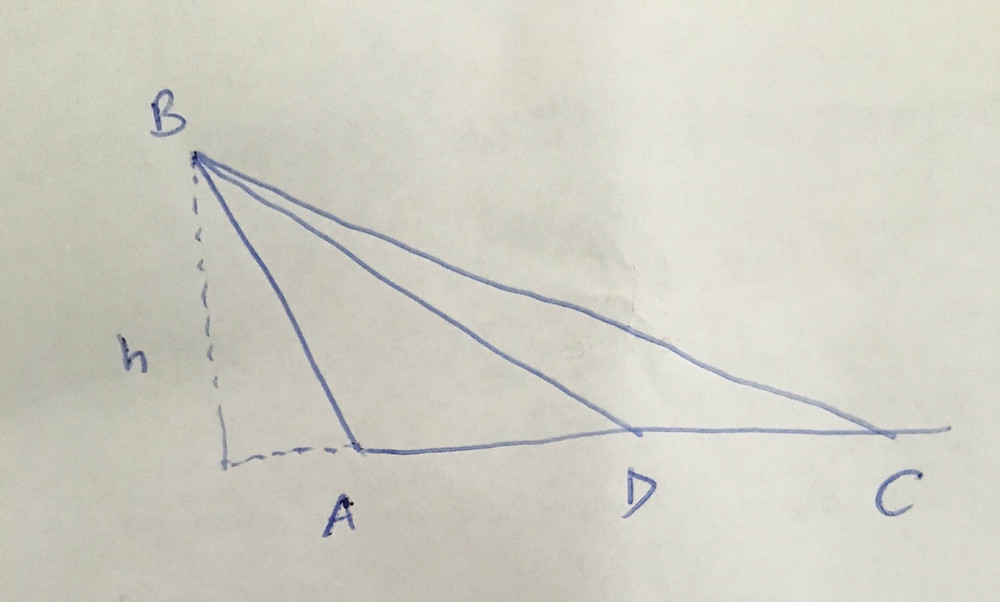# GMAT Quant question of the dayIn the figure above, D is a point on the side AC of ΔABC. Is ΔABC isosceles? (1) The area of the triangular region ABD is equal to the area of triangular region DBC. (2) BD is perpendicular to AC and AD = DC.

We are after: Is ΔABC isosceles?

(1) Using the formula for areas of triangle, we get

Area of ΔABD = Area of ΔABD 1/2 * AD * h = 1/2 * DC * hh is the height for both triangles, if you drop a perpendicular from point B on AC. This will fall on the extended AC (if you can imagine it)

The above equation yields to: AD = AC

But we want to know if ΔABC is isosceles. This information doesn't help so it is INSUFFICIENT.

(2)

Since BD ⊥AC, and D is on AC, imagine D dividing AC into two sides i.e. AD =AC.Now imagine two right angled Δs inside ABC being split in the middle by BD. These two right angled Δs have the same base and height so the hypotenuse will be same i.e. AB = AC.

Sufficient